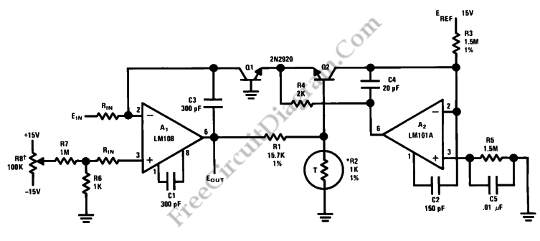# Log Converter with 100dB Dynamic RangeThis is a circuit of Log Converter with 100dB Dynamic Range. This circuit can give a a logarithmic output voltage for a linear input current. This circuit use an LM108 operational amplifier with Transistor Q1 as non-linear feedback element.  R1 and R2 are used as divider. Negative feedback is applied to the emitter base junction of Q2 and the emitter of Q1 through divider. Here is the circuit:This circuit has The temperature compensation that is typically 1% over a temperature range of 25’C to 100’C for the resistor specified. To set the gain, this circuit uses divider. The current through R3 sets the zero. A 430R sensistor in series with a 570R resistor should be replaced for the 1k resistor, also with 1% accuracy if the circuit is used for limited temperature range applications, such as 0’C to 50’C. [Source: National Semiconductor Application Note]